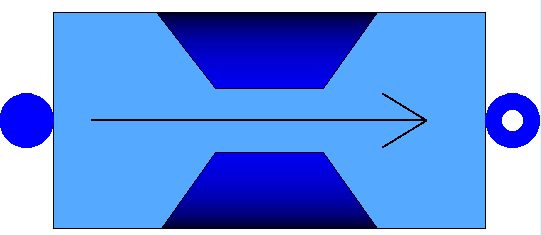# Information

The DP model is a lumped model that computes a punctual pressure drop.

The assumptions for this model are:

• Incompressible fluid for computing the pressure drop
• No thermal energy losses to the ambient

The total pressure drop is computed as the sum of three different terms: a constant pressure difference (e.g. due to the static pressure head), a linear pressure drop (e.g. due to friction in a laminar flow) and a quadratic pressure drop (typical of turbulent flow).

#Modelling options

In the General tab the following options are availabe:

• Medium: the user has the possibility to easly switch Medium.
• UseNom: If true, use the nominal conditions defined in the Nominal Conditions tab to compute the pressure drop characteristic (i.e. the parameters h, K and A)

In the Nominal condition tab the following option is availabe:

• use_rho_nom: If true, the density is considered constant during the whole simulation and equal to the nominal value.

In the Initialization tab the following options are availabe:

• constinit: If true, the pressure drop is considered constant at the beginning of the simulation to avoid oscillations.
• UseHomotopy: If true, the homotopy function is used to set the pressure drop to zero in the first initialization.

The parameter DELTAp_0 defines a limitation of the pressure drop below which the quadratic expression is replaced by a third order polynomial expression to avoid the non-physical infinite derivative at DELTAp=0.